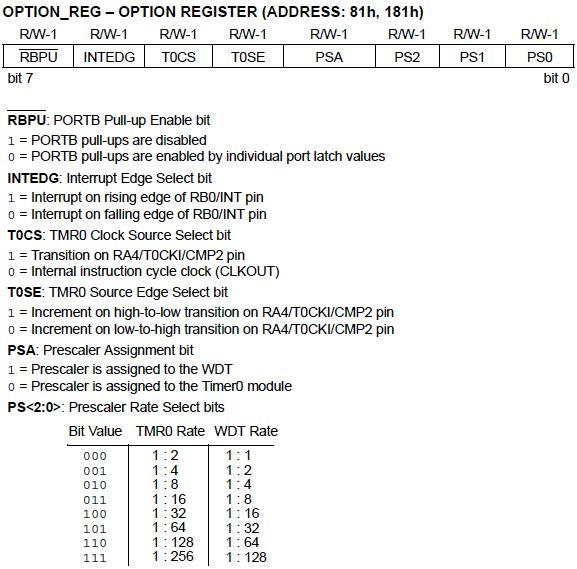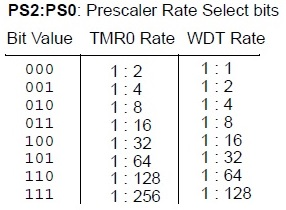# Using PIC Timer with PIC16F84A

In this tutorial, I will discuss how to use the PIC16F84A microcontroller timer module for a variety of applications including turning a LED on and off without the use of a software delay subroutine.

### Introduction

The PIC16F84A has one 8-bit free-running timer named TMR0. The term "free-running" means it continually runs on the background and has no start or stop control. You can read or write values from/to this timer. Since it's an 8-bit timer, the TMR0 can count from 0 to 255 (28 = 256).

Other microcontrollers have multiple timers with higher bit numbers: the PIC16F877A has two 8-bit and one 16-bit timers while the PIC18F4550 has three 16-bit and one 8-bit timers. Each count elapses after two instruction cycles by default. Recall that the instruction cycle speed is calculated (for a 4 MHz oscillator) using

You can extend the time it takes for each count using prescalers. When the timer reaches 255, the timer overflows and will go back to 0. An interrupt is associated with timer overflow.

### Configuring the PIC Timer

All settings for the TMR0 is configured using the OPTION register. The PIC16F84A datasheet describes this register as follows:

The OPTION register is a readable and writable register which contains various control bits to configure the TMR0/WDT prescaler, the external INT interrupt, TMR0, and the weak pull-ups on PORTB.

Here are the bits for this register:Look at bit 5 of the OPTION register named TOCS (TMR0 Clock Source Select). To use the timer, this bit should be cleared. If this is set, the TMR0 module is used for counting (discussed in a separate tutorial).

### Prescaler

The prescaler will determine how many source edges will increment the TMR0 register value by 1. In short, the prescaler allows us to extend the time before the timer overflows. There are eight prescale settings which are chosen via the PS <2:0> bits of the OPTION register:Bit 3 is the prescale assignment bit. Clearing it will assign the prescale to TMR0. Setting it would assign the prescale to the watchdog timer (which is another timer with a different use). Note that any write instructions to TMR0 (clrf, bsf, movwf, etc) will clear the prescale value.

Example: If the prescaler if set to the TMR0 and PS <3:0> is binary 2 (0102), then it would take 8 counts before the timer increments by one and so overflows after 2.04 milliseconds (assuming a 4 MHz crystal is used) because

### Setting up the PIC Timer via ASM

Here is a short code snippet that configures the timer using the maximum prescale.

### Turning a LED On/Off using PIC Timer

What this code does is simply toggle the state of PORTB.0 synchronously with bit 0 of the TMR0 register. The toggling is a bit fast since the least significant bit changes state the fastest (around 254 microseconds for a 4 MHz crystal) for every count.

To make it slower, you can use the next bit (bit 1) by changing

to

Of course, PORTB.1 will now be blinking instead of PORTB.0. The slowest blink can be achieved if andlw 0x80 (masking the most significant bit) is used.

### A One Second Delay

So how can we achieved a delay of one second? If you do the math, the timer overflows (max prescale, 4 MHz crystal) after 65.536 milliseconds.  That's around 1/15 of a full second. Knowing this, we can wait for the timer to overflow 15 times before toggling the state of a pin. This is achieved by the code below:

What I did here is continually subtract 255 from TMR0.  If TMR0 reaches 255, the result of the sublw .255 instruction would be zero, setting the Z bit of the STATUS register. When this happens, the COUNT variable which is initialized to 15, will be decreased by 1. PORTB.0 will be set when the COUNT variable reaches zero. The process is then repeated to delay the clearing of PORTB.0.

Although the above code works, I still suggest you use the timer overflow interrupt to delay things which uses less program space.

Next>> Asynchronous Serial (USART) Communication with PIC16F877A